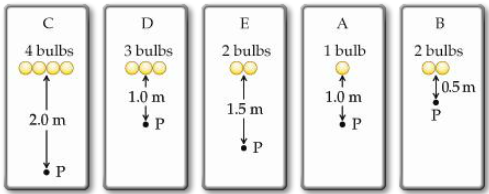# Problem: Rank the light intensity, from largest to smallest, at the point P in the figures.

###### FREE Expert Solution

Light intensity, I:

$\overline{){\mathbf{I}}{\mathbf{=}}{\mathbf{n}}\frac{\mathbf{P}}{\mathbf{4}\mathbf{\pi }{\mathbf{r}}^{\mathbf{2}}}}$###### Problem Details

Rank the light intensity, from largest to smallest, at the point P in the figures.